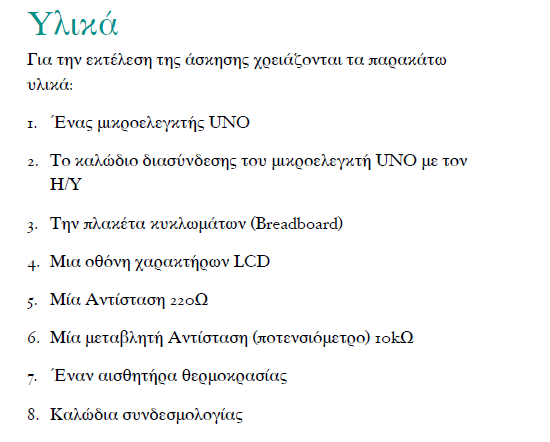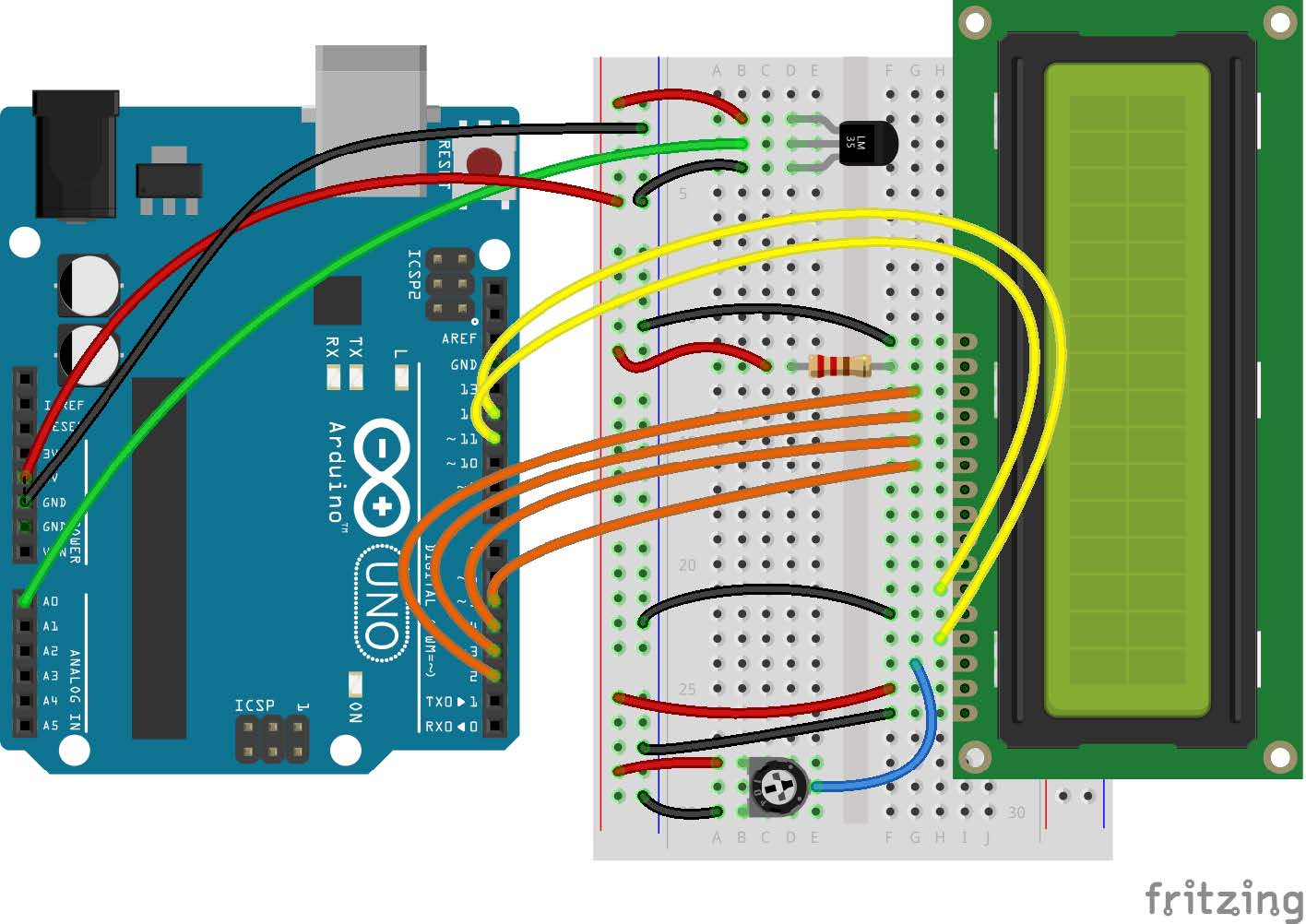tel. 24910 23755 / fax 24910 26620`/*`
`Display temperature in an LCD display`
`in celsius`
`*/`
`// include LCD library headers`
`#include <LiquidCrystal.h>`
`// constants won't change. They're used here to`
`// set pin numbers:`
`// in Pin 0 we will connect the temperature`
`// sensor. Give it a name.`
`const int tempPin = 0;`
`// variables will change:`
`// variable for reading the temperature from`
`// sensor`
`int tempReading;`
`// variables to convert sensor reading to C`
`float tempVolts, tempC;`
`// setup LCD`
`// RS E D4 D5 D6 D7`
`LiquidCrystal lcd(12, 11, 5, 4, 3, 2);`
`// the setup routine runs once when you press`
`// reset:`
`void setup() {`
`  // initialize the LCD`
`  lcd.begin(16, 2);`
`  lcd.setCursor(0, 1);`
`  lcd.print("Room condition");`
`  lcd.setCursor(0, 0);`
`  lcd.print("Temp C: ");`
`}`
`// the loop routine runs over and over again`
`// forever:`
`void loop() {`
`  // read the temperature`
`  tempReading = analogRead(tempPin);`
`  // convert reading to C`
`  tempVolts = tempReading * 5.0 / 1024.0;`
`  tempC = (tempVolts - 0.5) * 100.0;`
`  // print it to LCD`
`  lcd.setCursor(8, 0);`
`  lcd.print(tempC);`
`  delay(500);`
`}`

Κατηγορίες: Arduino